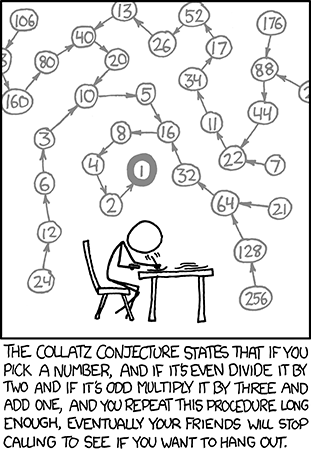# Collatz Conjecture — What You Need to Know

Like many of my previous posts, this post too has something to do with a Project Euler problem. Here’s a sketch of the Colatz Conjecture.

The following iterative sequence is defined for the set of positive integers:

n → n/2 (n is even)
n → 3n + 1 (n is odd)

Using the rule above and starting with 13, we generate the following sequence:

13 → 40 → 20 → 10 → 5 → 16 → 8 → 4 → 2 → 1

So basically, it’s just this. Take any natural number n. If n is even, divide it by 2 to get n / 2. If n is odd, multiply it by 3 and add 1 to obtain 3n + 1. Repeat the process indefinitely. The conjecture is that no matter what number you start with, you will always eventually reach 1. The property has aptly been called oneness! But perhaps oneness has its pitfalls too…If the conjecture is false, it can only be because there is some starting number which gives rise to a sequence that does not contain 1. Such a sequence might enter a repeating cycle that excludes 1, or increase without bound. No such sequence has been found.

Question
It can be seen that the sequence:
13 → 40 → 20 → 10 → 5 → 16 → 8 → 4 → 2 → 1
contains 10 terms. Although it has not been proved yet (Collatz Problem), it is thought that all starting numbers finish at 1. Which starting number, under one million, produces the longest chain?

NOTE: Once the chain starts the terms are allowed to go above one million.

HUGE HINT:

Histogram of stopping times for the numbers 1 to 100 million. Stopping time is on the x axis, frequency on the y axis.Approach 1 (A naïve, but straigh forward method)

 # Longest Collatz Sequence under a million # Function listing collatz sequence for a number def collatz(n): "function listing collatz sequence for a positive integer" coll = [] coll.append(n) while n != 1: if n % 2 == 0: n = n/2 coll.append(n) else: n = 3*n + 1 coll.append(n) return coll longest = 0 j = 0 for i in xrange(1, 1000000): lencoll = len(collatz(i)) if lencoll > longest: longest = lencoll j = i print j
view raw euler14.py hosted with ❤ by GitHub

Approach 2 (Smart, quick method that uses dynamic programming with the help of dictionaries)

 collatz = {1:1} def Collatz(n): global collatz if not collatz.has_key(n): if n%2 == 0: collatz[n] = Collatz(n/2) + 1 else: collatz[n] = Collatz(3*n + 1) + 1 return collatz[n] for j in range(1000000,0,-1): Collatz(j) print collatz.keys()[collatz.values().index(max(collatz.values()))]
view raw euler14.py hosted with ❤ by GitHub

I couldn’t help appreciate the elegance of the second algorithm. It’ll be well worth perusing if you don’t get it at one go. [Hint: It keeps track of the number of terms of a particular sequence as values assigned to keys of a Python dictionary]

Ans: 837799